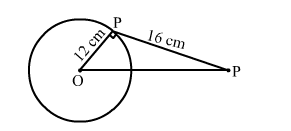# Assertion (A) At point P of a circle with centre O and radius 12 cm,`
Question:

Assertion (A)
At point P of a circle with centre O and radius 12 cm, a tangent PQ of length 16 cm is drawn. Then, OQ = 20 cm.

Reason (R)
The tangent at any point of a circle is perpendicular to the radius through the point of contact.

(a) Both Assertion (A) and Reason (R) are true and Reason (R) is a correct explanation of Assertion (A).
(b) Both Assertion (A) and Reason (R) are true but Reason (R) is not a correct explanation of Assertion (A).
(c) Assertion (A) is true and Reason (R) is false.
(d) Assertion (A) is false and Reason (R)is true.

Solution:(a) Both Assertion (A) and Reason (R) are true and Reason (R) is a correct explanation of Assertion (A).

In $\Delta O P Q, \angle O P Q=90^{\circ}$.

$\therefore O Q^{2}=O P^{2}+P Q^{2}$

$\Rightarrow O Q=\sqrt{O P^{2}+P Q^{2}}$

$=\sqrt{12^{2}+16^{2}}$

$=\sqrt{144+256}$

$=\sqrt{400}$

$=20 \mathrm{~cm}$